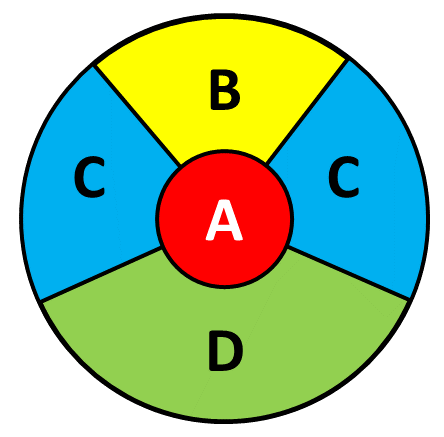# Exam-Style Questions.

## Problems adapted from questions set for previous Mathematics exams.

### 1.

GCSE Higher

There are only crayons in a pencil case and the crayons are either orange, purple or brown.

The table shows the probability of taking at random a brown crayon from the pencil case.

 Colour: Probability: orange purple brown 0.4

The number of orange crayons in the pencil case is the same as the number of purple crayons.

(a) Complete the table.

There are 20 brown crayons in the pencil case.

(b) Work out the total number of crayons in the pencil case.

### 2.

GCSE Higher

The following table shows the probabilities of winning certain prizes at a stall in Faulton Towers theme park. A prize is always given for every turn.

 Prize Probability Pencil Calculator Teddy Bear Ball Protractor 0.65 0.02 0.05 0.26 ?

In the last year Simon has had 200 turns receiving 200 prizes.

Work out an estimate for the total number of times he has won either a pencil or a protractor.

### 3.

GCSE Higher

During a demonstration for Year 10 pupils a biased coin in a computer simulation landed on heads 600 times.

The relative frequency of heads was 0.3

Work out the number of times the coin landed on tails during that demonstration.

### 4.

GCSE Higher

A bag contains balls that are red, blue, green or yellow.

• The number of red balls is $$x-3$$.
• The number of blue balls is $$2x$$.
• The number of green balls is $$7$$.
• The number of yellow balls is $$5x$$.

A counter is chosen at random. The probability it is green is $$\frac{7}{52}$$

Work out the probability it is red.

### 5.

IB Standard

A game at a fayre consists of a players throwing one dart at the board pictured below.The probability of hitting each region and the points scored for hitting that region is given in this table.

RegionProbabilityPoints
A$$\frac{1}{25}$$50
B$$\frac{2}{25}$$$$x$$
C$$\frac{4}{25}$$20
D$$\frac{5}{25}$$10

(a) Find the probability that the dart does not hit the board.

The player scores points as shown in the table above but they lose 20 points if they miss the board completely.

(b) Given that the game is a fair game, find the value of $$x$$, the number of points awarded for hitting the B region of the board.

If you would like space on the right of the question to write out the solution try this Thinning Feature. It will collapse the text into the left half of your screen but large diagrams will remain unchanged.

The exam-style questions appearing on this site are based on those set in previous examinations (or sample assessment papers for future examinations) by the major examination boards. The wording, diagrams and figures used in these questions have been changed from the originals so that students can have fresh, relevant problem solving practice even if they have previously worked through the related exam paper.

The solutions to the questions on this website are only available to those who have a Transum Subscription.

Exam-Style Questions Main Page

Search for exam-style questions containing a particular word or phrase:

To search the entire Transum website use the search box in the grey area below.